SYLLABUS  Previous: 1.4.4 Splines  Up: 1.4 Numerical discretization  Next: 1.4.6 Wavelets

### 1.4.5 Harmonic functions

A harmonic decomposition is obtained from a discrete Fourier transform (DFT) assuming a regular mesh and a periodic domain of length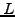. Using the notations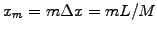and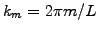, the forward and backward transformations are defined as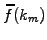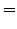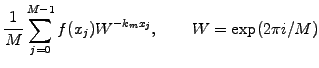(1.4.5#eq.1)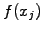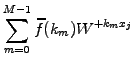(1.4.5#eq.2)

If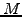is a power of 2, the total number of operations can be dramatically reduced from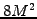to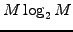in a fast Fourier transform (FFT), by applying recursively the decomposition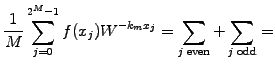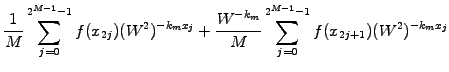with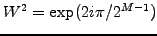until a sum of DFT of length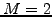is obtained. Figure (1.4.5#fig.1) illustrates how the approximation of a periodic square wave converges with an increasing number of Fourier components. Note how the function overshoots in the neighborhood of sharp edges: this is the Gibbs phenomenon and it will always be there for a finite number of terms in the sum.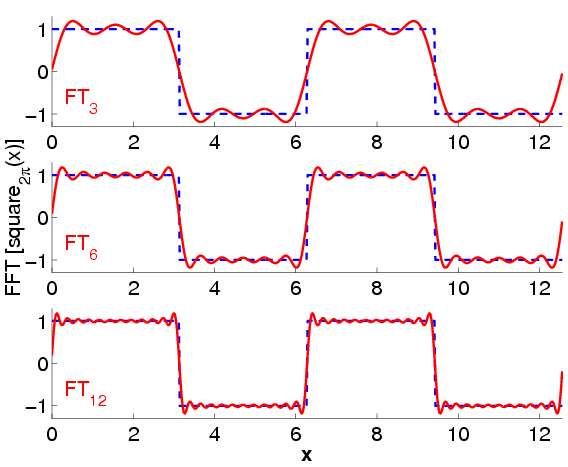It should not be surprizing to hear that a harmonic decomposition is particularly well suited for smooth global functions with long wavelengths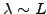, since they result in a narrow spectrum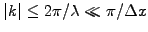. Note that the convergence with an increasing number of Fourier components is faster than any polynomial once the smallest structure has been resolved. The implementation of non-periodic boundary conditions, however, can sometimes be problematic.

SYLLABUS  Previous: 1.4.4 Splines  Up: 1.4 Numerical discretization  Next: 1.4.6 Wavelets